Next: Magnetic Susceptibility and Permeability Up: Magnetostatics in Magnetic Media Previous: Magnetostatics in Magnetic Media

# Magnetization

All matter is built up out of atoms, and every atom contains moving electrons. The currents associated with these electrons are termed atomic currents. Each atomic current is a tiny closed circuit of atomic dimensions, and may therefore be appropriately described as a magnetic dipole. If the atomic currents of a given atom all flow in the same plane then the atomic dipole moment is directed normal to the plane (in the sense given by the right-hand circulation rule), and its magnitude is the product of the total circulating current and the area of the current loop. More generally, if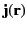is the atomic current density at point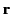then the magnetic moment of the atom is [see Equation (676)]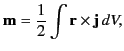(680)

where the integral is over the volume of the atom. If there are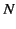such atoms or molecules per unit volume then the magnetization,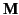, (i.e., the magnetic dipole moment per unit volume) is given by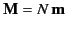. More generally,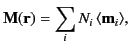(681)

where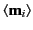is the average magnetic dipole moment of the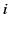th type of molecule in the vicinity of point, and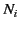is the average number of such molecules per unit volume at.

Consider a general medium that is made up of molecules that are polarizable, and possess a net magnetic moment. It is easily demonstrated that any circulation in the magnetization field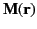gives rise to an effective current density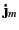in the medium. In fact,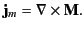(682)

This current density is called the magnetization current density, and is usually distinguished from the true current density,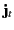, which represents the convection of free charges in the medium. In fact, there is a third type of current called a polarization current, which is due to the apparent convection of bound charges. It is easily demonstrated that the polarization current density,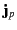, is given by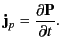(683)

Thus, the total current density,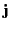, in the medium is given by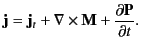(684)

It must be emphasized that all three terms on the right-hand side of the previous equation represent real physical currents, although only the first term is due to the motion of charges over more than atomic dimensions.

The fourth Maxwell equation, (4), takes the form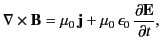(685)

which can also be written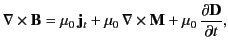(686)

where use has been made of the definition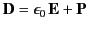. The previous expression can be rearranged to give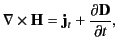(687)

where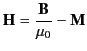(688)

is termed the magnetic intensity, and has the same dimensions as(i.e., magnetic dipole moment per unit volume). In a steady-state situation, the curl theorem tell us that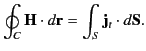(689)

In other words, the line integral of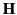around some closed loop is equal to the flux of true current through any surface attached to that loop. Unlike the magnetic field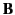(which specifies the force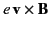acting on a chargemoving with velocity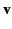), or the magnetization(which specifies the magnetic dipole moment per unit volume), the magnetic intensityhas no clear physical meaning. The only reason for introducing it is that it enables us to calculate magnetic fields in the presence of magnetic materials without first having to know the distribution of magnetization currents. However, this is only possible if we possess a constitutive relation connectingand.Next: Magnetic Susceptibility and Permeability Up: Magnetostatics in Magnetic Media Previous: Magnetostatics in Magnetic Media
Richard Fitzpatrick 2014-06-27